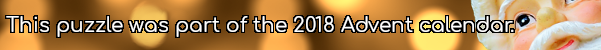mscroggs.co.uk
mscroggs.co.uksubscribe

# Puzzles

## Not Roman numerals

The letters $$I$$, $$V$$ and $$X$$ each represent a different digit from 1 to 9. If
$$VI\times X=VVV,$$
what are $$I$$, $$V$$ and $$X$$?

## 24 December1,0,2,0,1,1
The sequence of six numbers above has two properties:
1. Each number is either 0, 1 or 2.
2. Each pair of consecutive numbers adds to (strictly) less than 3.
Today's number is the number of sequences of six numbers with these two properties
Tags: numbers

## 22 DecemberIn base 2, 1/24 is 0.0000101010101010101010101010...
In base 3, 1/24 is 0.0010101010101010101010101010...
In base 4, 1/24 is 0.0022222222222222222222222222...
In base 5, 1/24 is 0.0101010101010101010101010101...
In base 6, 1/24 is 0.013.
Therefore base 6 is the lowest base in which 1/24 has a finite number of digits.
Today's number is the smallest base in which 1/10890 has a finite number of digits.
Note: 1/24 always represents 1 divided by twenty-four (ie the 24 is written in decimal).

## 21 DecemberPut the digits 1 to 9 (using each digit exactly once) in the boxes so that the sums are correct. The sums should be read left to right and top to bottom ignoring the usual order of operations. For example, 4+3×2 is 14, not 10. Today's number is the smallest number you can make using the digits in the red boxes.
 + ÷ = 2 × + - × - = 31 + + - - × = 42 =37 =13 =-2
Tags: numbers, grids

## 20 DecemberToday's number is the sum of all the numbers less than 40 that are not factors of 40.

## 17 DecemberFor $$x$$ and $$y$$ between 1 and 9 (including 1 and 9), I write a number at the co-ordinate $$(x,y)$$: if $$x\lt y$$, I write $$x$$; if not, I write $$y$$.
Today's number is the sum of the 81 numbers that I have written.
Tags: numbers

## 16 DecemberArrange the digits 1-9 in a 3×3 square so that the first row makes a triangle number, the second row's digits are all even, the third row's digits are all odd; the first column makes a square number, and the second column makes a cube number. The number in the third column is today's number.
 triangle all digits even all digits odd square cube today's number
Tags: numbers, grids

## 15 DecemberToday's number is smallest three digit palindrome whose digits are all non-zero, and that is not divisible by any of its digits.

## Archive

Show me a random puzzle
▼ show ▼
© Matthew Scroggs 2019